# Zero Rh

Select the Zero Rh product you are looking for or choose a different brand

Select the brand alphabetically

Sort by
Show Next 50
Showing 1-50 of 93Zero Rh + V Womens Be the First! WRITE A REVIEW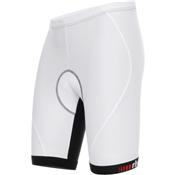Zero Rh + Prime Be the First! WRITE A REVIEWZero Rh + Aria Leg Be the First! WRITE A REVIEWZero Rh + Agility Womens Be the First! WRITE A REVIEW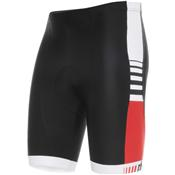Zero Rh + Legend Be the First! WRITE A REVIEWZero Rh + Logo Leg Be the First! WRITE A REVIEWZero Rh + V Sleeveless Womens Be the First! WRITE A REVIEWZero Rh + Corporate Bag II Be the First! WRITE A REVIEWZero Rh + Comet FullZip Sleeve Wo Be the First! WRITE A REVIEW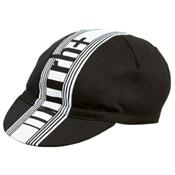Zero Rh + Zero Cap Be the First! WRITE A REVIEWZero Rh + Fusion Sleeve Wo Be the First! WRITE A REVIEWZero Rh + Powerlogic Be the First! WRITE A REVIEW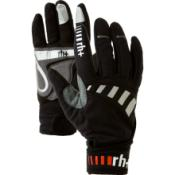Zero Rh + Ergo Be the First! WRITE A REVIEWZero Rh + Grand Prix LongSleeve Be the First! WRITE A REVIEWZero Rh + Dynamic Be the First! WRITE A REVIEWZero Rh + Class Be the First! WRITE A REVIEWZero Rh + Leader Sleeve Be the First! WRITE A REVIEWZero Rh + Ergo 6 Be the First! WRITE A REVIEWZero Rh + Ergo HalfFinger Be the First! WRITE A REVIEWZero Rh + Air Wind Long Sleeve Be the First! WRITE A REVIEWZero Rh + Ergo 13 Be the First! WRITE A REVIEWZero Rh + Racer Be the First! WRITE A REVIEW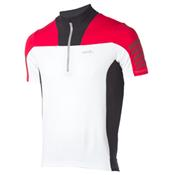Zero Rh + Canyon Be the First! WRITE A REVIEW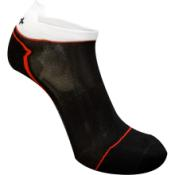Zero Rh + Micro Be the First! WRITE A REVIEWZero Rh + Team Be the First! WRITE A REVIEW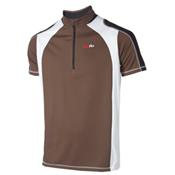Zero Rh + Mesa Verde Be the First! WRITE A REVIEWZero Rh + Flux Womens Be the First! WRITE A REVIEW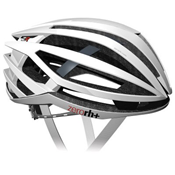Zero Rh + ZR Be the First! WRITE A REVIEW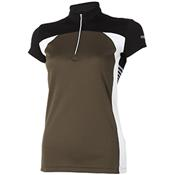Zero Rh + Mesa Verde Women's Be the First! WRITE A REVIEW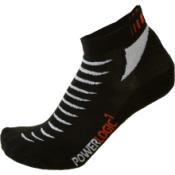Zero Rh + Powerlogic Sock Be the First! WRITE A REVIEW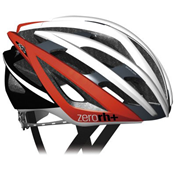Zero Rh + ZX Be the First! WRITE A REVIEW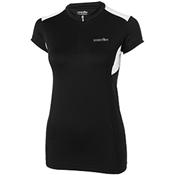Zero Rh + Powerlogic Ride Women's Be the First! WRITE A REVIEW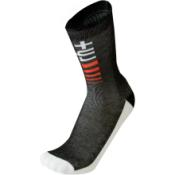Zero Rh + Ergo Be the First! WRITE A REVIEW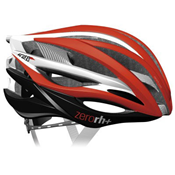Zero Rh + ZW Be the First! WRITE A REVIEW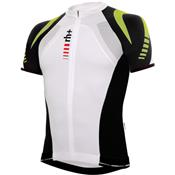Zero Rh + ZeeKey S/S Be the First! WRITE A REVIEW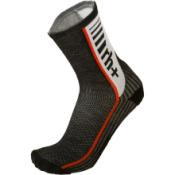Zero Rh + Dynamic Sock Be the First! WRITE A REVIEW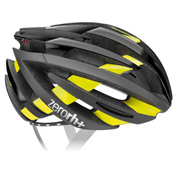Zero Rh + ZY Be the First! WRITE A REVIEW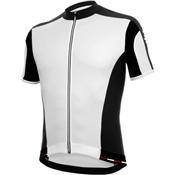Zero Rh + Vertigo S/S Be the First! WRITE A REVIEW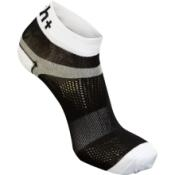Zero Rh + Ergo 6 Sock Be the First! WRITE A REVIEW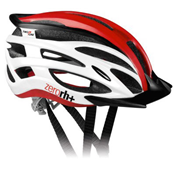Zero Rh + Two in One Be the First! WRITE A REVIEW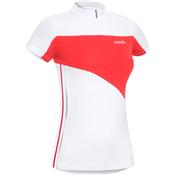Zero Rh + Up & Down S/S Women's Be the First! WRITE A REVIEW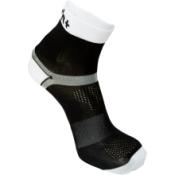Zero Rh + Ergo 13 Sock Be the First! WRITE A REVIEW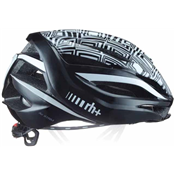Zero Rh + Air XTRM Be the First! WRITE A REVIEW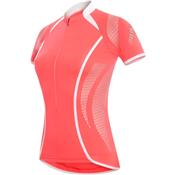Zero Rh + Stretch Control S/S Women's Be the First! WRITE A REVIEWZero Rh + Micro Sock Be the First! WRITE A REVIEW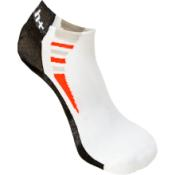Zero Rh + Flux Sock - Women''''s Be the First! WRITE A REVIEW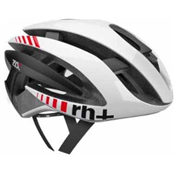Zero Rh + Z Alpha Mips Be the First! WRITE A REVIEW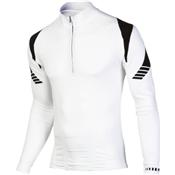Zero Rh + Key-Jey L/S Be the First! WRITE A REVIEWZero Rh + Ergo 9 Be the First! WRITE A REVIEW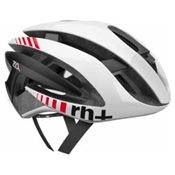Zero Rh + Z Alpha Be the First! WRITE A REVIEW
Show Next 50
Showing 1-50 of 93